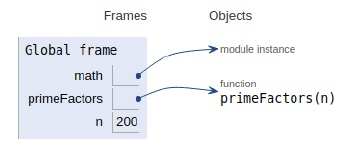# Python Program for Efficient program to print all prime factors of a given number

Problem statement − We are given a number, we need to find all the prime factors of a given number.

The efficient solution to the problem is discussed below −

## Example

Live Demo

# Python program to print prime factors
import math
# prime
def primeFactors(n):
# no of even divisibility
while n % 2 == 0:
print (2),
n = n / 2
# n reduces to become odd
for i in range(3,int(math.sqrt(n))+1,2):
# while i divides n
while n % i== 0:
print (i)
n = n / i
# if n is a prime
if n > 2:
print (n)
n = 200
primeFactors(n)

## Output

2
2
2
5
5All the variables and functions are declared in the global scope as shown in the figure above.

## Conclusion

In this article, we have learned how we can print all prime factors of a given number efficiently.